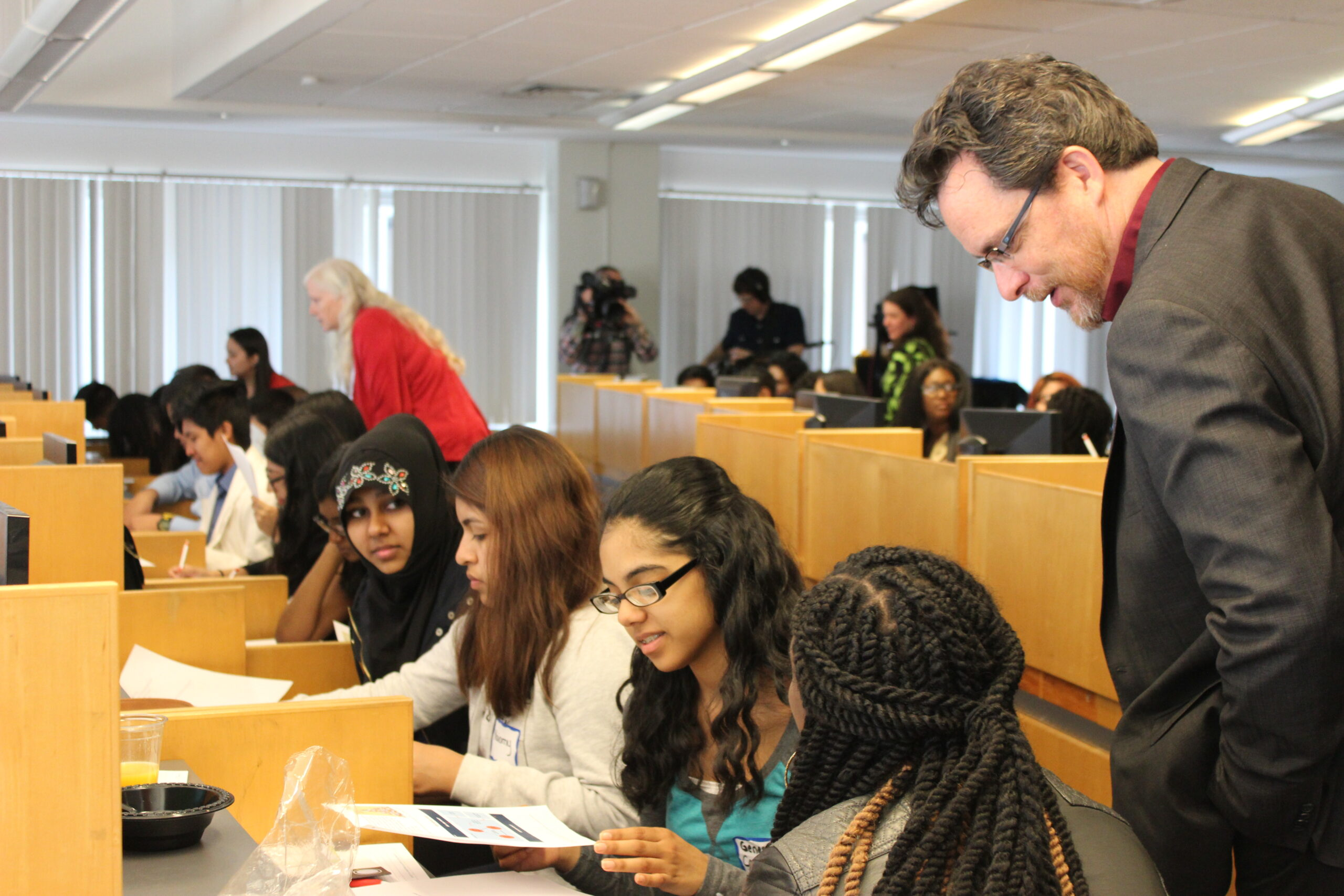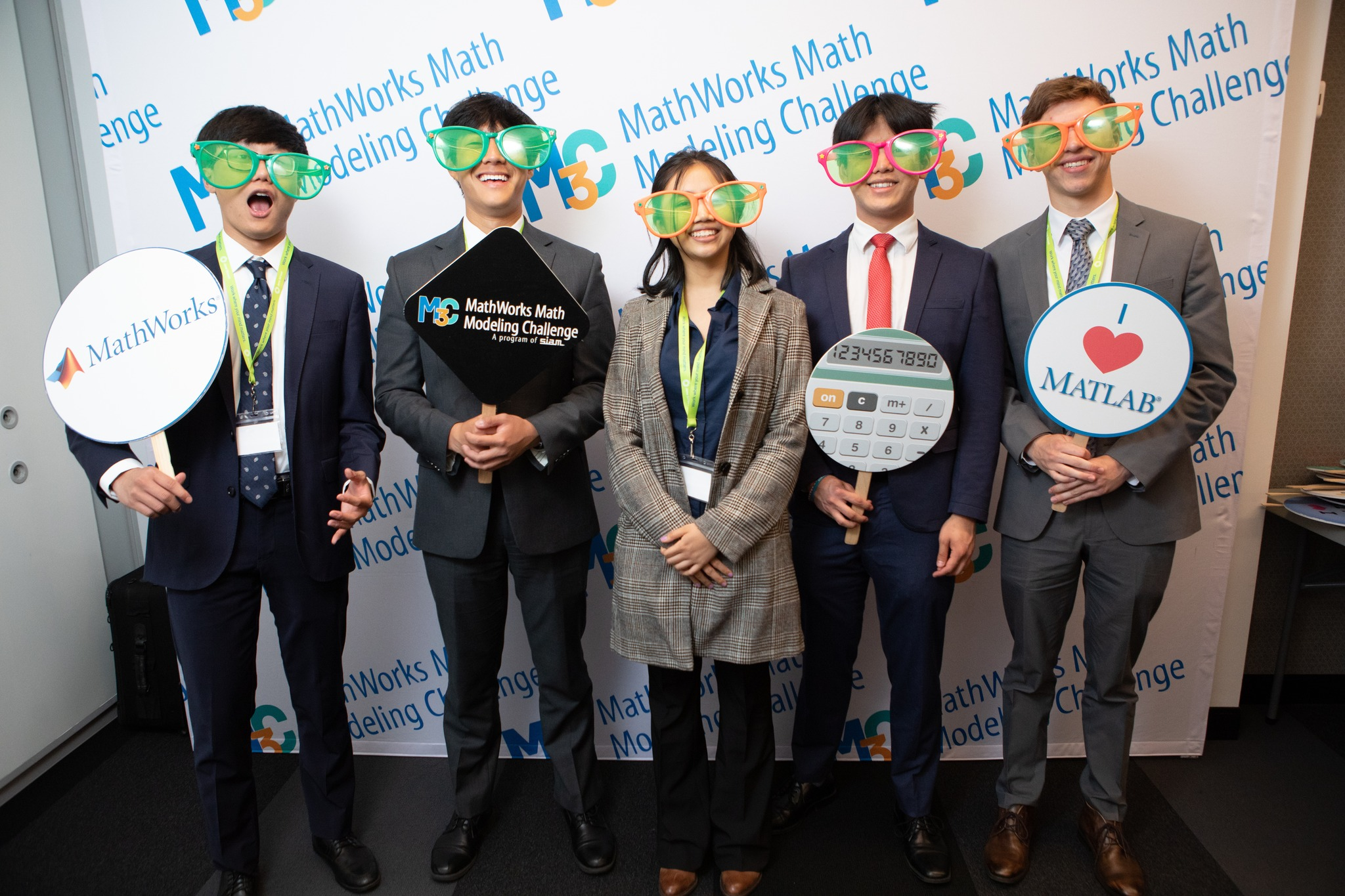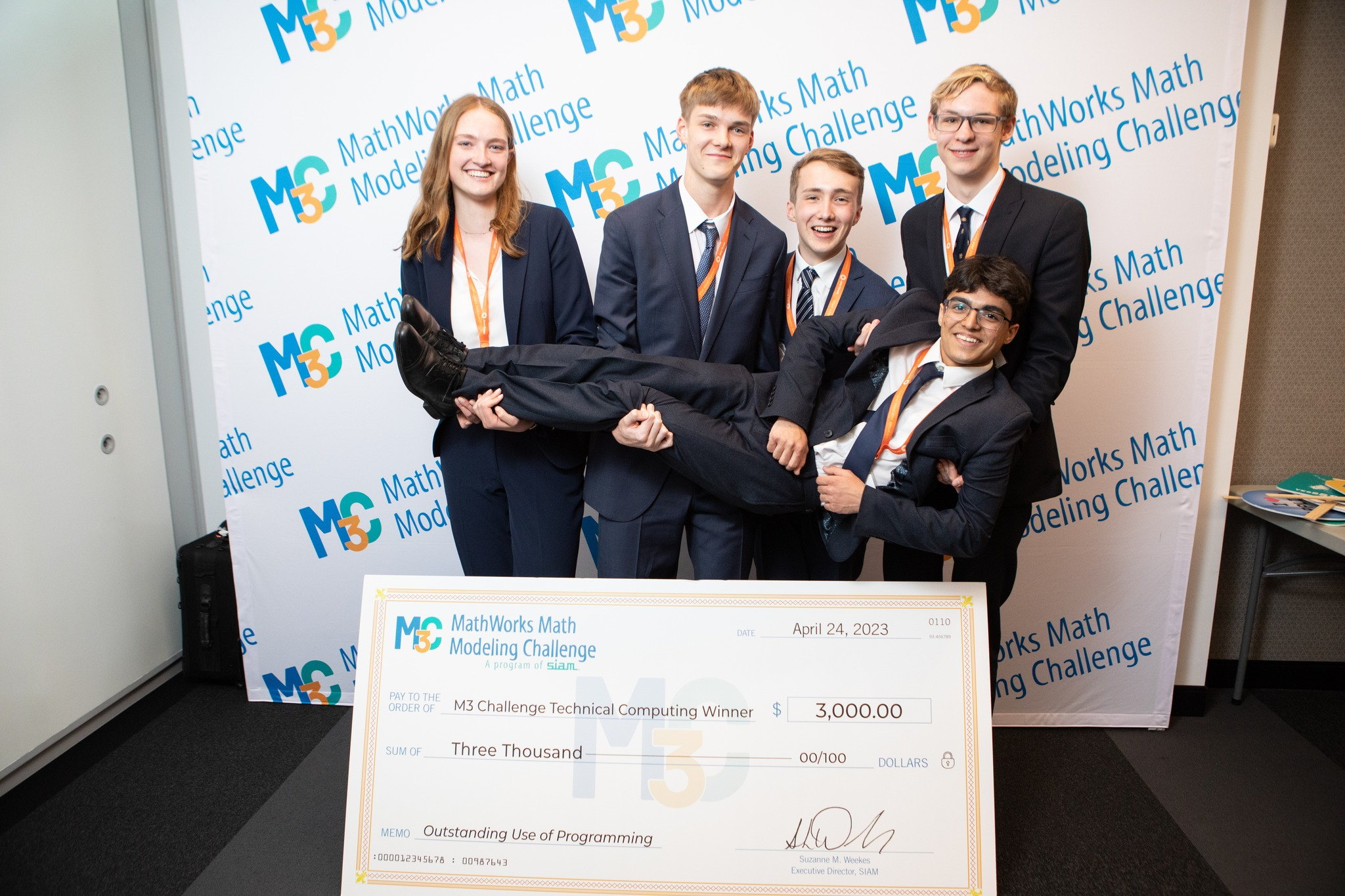## Learn Technical Computing

Technical Computing is the application of mathematical and computational principles to solve practical real-world problems.## What is MATLAB?

• Import and analyze data
• Develop mathematical models
• Visualize data and share results

## Learn MATLAB techniques with these tutorials:

Learn how to solve a linear regression problem with MATLAB®. Follow a typical linear regression workflow and learn how you can interactively train, validate, and tune different models using the Regression Learner app.

Learn how to perform curve fitting in MATLAB® using the Curve Fitting app, and fit noisy data using smoothing spline. This video shows you how to use the Curve Fitting app to interactively try a variety of fitting algorithms, assess the fit numerically, and generate code from the app.

MATLAB® has various functions that can be used to display data graphically. This video shows how to use some of the data distribution plots such as histograms, pie charts and heatmaps to display data. Interactively create and explore visualizations for your data using the Create Plot Live Editor task.

Learn how to insert equations into your Live Script to describe a mathematical process or method used in your code. This video shows you how to insert and generate LaTeX equations in MATLAB®.

Resources

## Get Started with MATLAB

### Get started with MATLAB

A guide to learn how to access MATLAB, learn how to code through free resources, and more!

### Teaching with MATLAB and Simulink?

Tasks designed for students in Years 10-12.

MATLAB and Simulink courseware in engineering, mathematics, programming, and robotics.

### MATLAB Cody

A game to sharpen your programming skills, solve problems and interact with the MATLAB community. Chances to win prizes!

### Winning Solutions Using MATLAB

See how winning teams use MATLAB in their solutions.